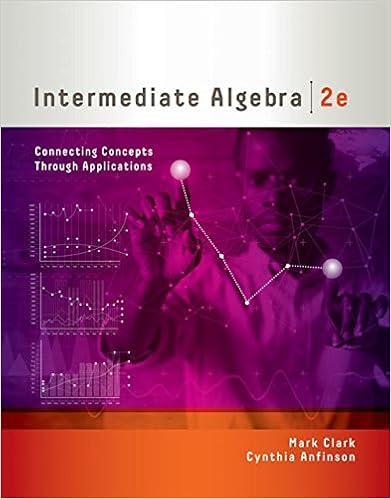• Test Prep
• 26
• 100% (72) 72 out of 72 people found this document helpful

This preview shows page 7 - 16 out of 26 pages.

##### We have textbook solutions for you!
The document you are viewing contains questions related to this textbook.The document you are viewing contains questions related to this textbook.
Chapter 1 / Exercise 83
Intermediate Algebra: Connecting Concepts through Applications
ClarkExpert Verified
b. c. d. The correct answer is:
##### We have textbook solutions for you!
The document you are viewing contains questions related to this textbook.The document you are viewing contains questions related to this textbook.
Chapter 1 / Exercise 83
Intermediate Algebra: Connecting Concepts through Applications
ClarkExpert Verified
4/27/2018Graded Midterm Exam8/26Question9Incorrect0.00 points outof 3.75Question10Suppose f(x) = x. What is the equation of the line tangent to the curve at the point (−1, −1)?3Incorrect0.00 points outof 3.75
4/27/2018Graded Midterm Exam9/26QuestionEvaluate the following as true or false. 11Correct3.75 points outof 3.75Question12Incorrect0.00 points outof 3.75
The correct answer is: falseSelect one:a. b. c. d.
4/27/2018Graded Midterm Exam10/26Question13Correct3.75 points outSelect one:a. b. c. d. The correct answer is:
of 3.75
4/27/2018Graded Midterm Exam11/26Question14What is the limit of the function in the graph at x= 4? Correct3.75 points outof 3.75Select one:a. 4b. 2c. 6d. The limit does not exist.The correct answer is: 2
4/27/2018Graded Midterm Exam12/26Question15Correct3.75 points outof 3.75Question16
0.00 points outof 3.75What is the equation of the line tangent to the curve y+ e= 2eat the point (0, 1)?Select one:a. y= xb. y= −x+ 1c. y= x− 1d. y= x+ 1
x
•••